# Exponential Graphs IV. Logarithm Graphs y=loght or y=lnx Domain: Domain: Range: Range: Intercept: y-intercept Find the...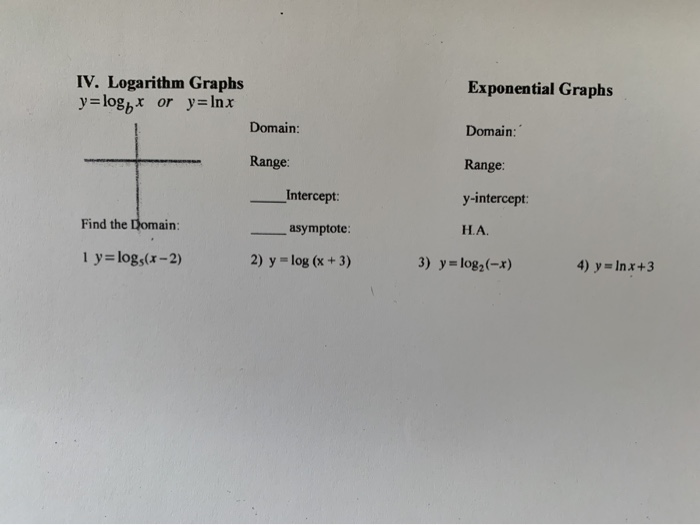Exponential Graphs IV. Logarithm Graphs y=loght or y=lnx Domain: Domain: Range: Range: Intercept: y-intercept Find the Domain: asymptote: HA 1 y=logs(x-2) 2) y = log (x+3) 3) y = log2 (-x) 4) y = Inx+3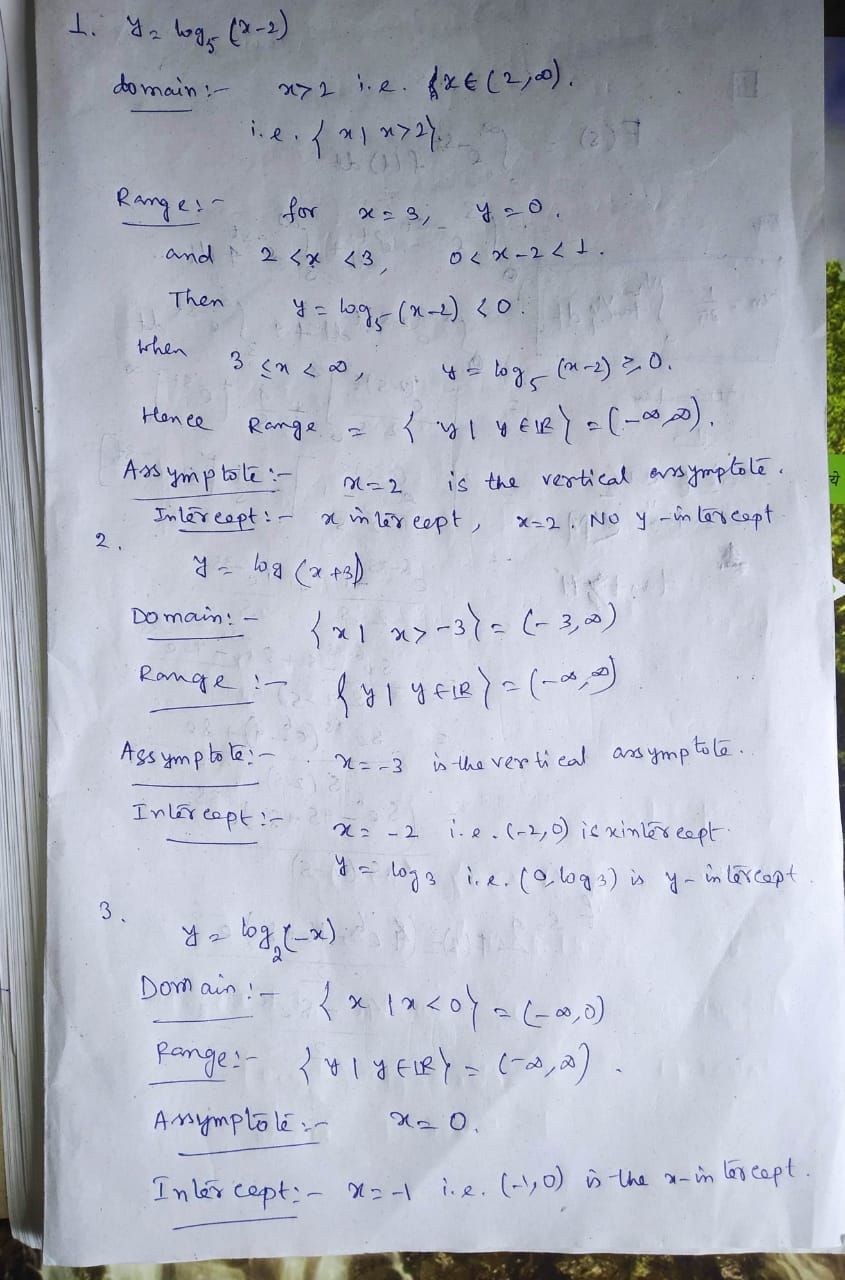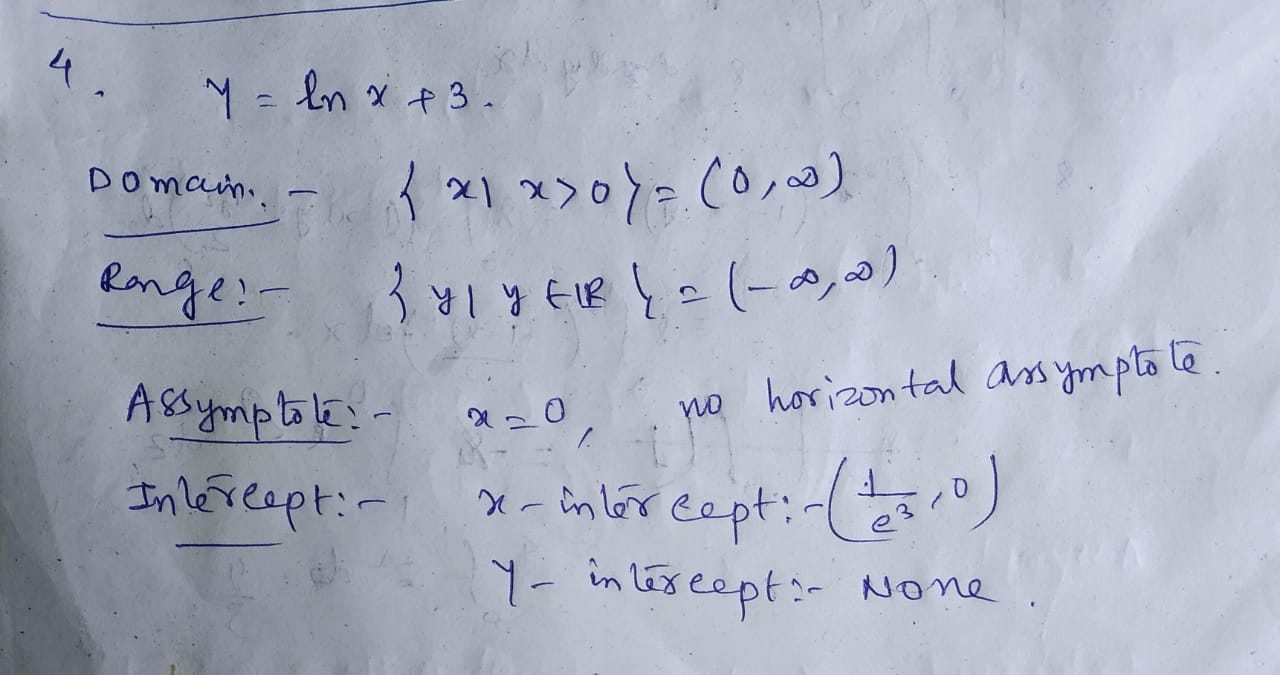##### Add Answer of: Exponential Graphs IV. Logarithm Graphs y=loght or y=lnx Domain: Domain: Range: Range: Intercept: y-intercept Find the...
Similar Homework Help Questions
• ### State the domain, range, x-intercept(s), y-intercept(s) and asymptote(s) of the given function.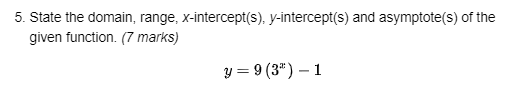State the domain, range, x-intercept(s), y-intercept(s) and asymptote(s) of the given function.  https://i.gyazo.com/ad9395dfa1f76d9e60f1346b476bc1ad.png

• ### holes, asymptopes, zeros, y intercept, domain, range

y= (x^2 + 3x - 4)/ x+4I have to find the holes, asymptopes, zeros, y intercept, domain, rangeAND I am totally clueless. I so don't get this....

• ### Express as a single logarithm

Express as a single logarithm. 1. logc t+logc s 2. (loga m-loga n Solve the equations. 1. log4x=log2+log(x-1) 2. log(3+x)-log(x-4)=log 2 3. log2(3x-2)-log2(x-5)=4 4. logx(8/27)=3

• ### Question: find the domain, vertical asymptote, and the x-intercept of f(x)=log(subscript 4)(5-x)

Question: find the domain, vertical asymptote, and the x-intercept of f(x)=log(subscript 4)(5-x).

• ### EGR MA / Wins Web IV 6. (5 points each) Find the domain and range for...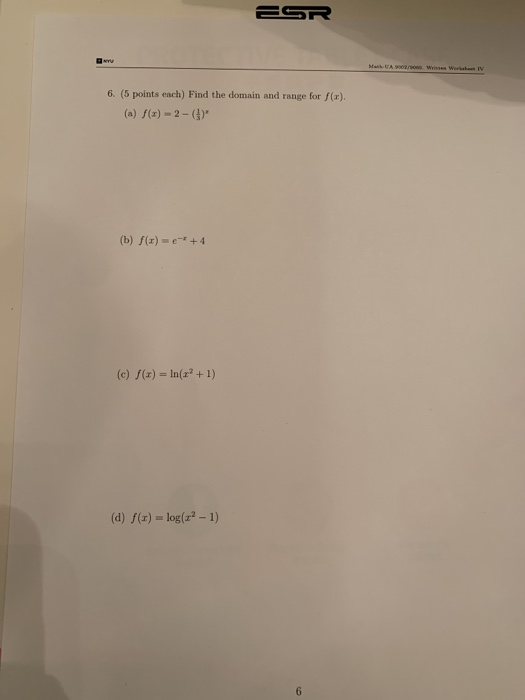EGR MA / Wins Web IV 6. (5 points each) Find the domain and range for f(r). (a) f(x) -2-(1) (b) f(x) = - +4 (c) f(x) = In(x+1) (d) f(x) = log(x2 - 1) 6

• ### 2. (10 points) is the below graph a function? If so find the Domain, Range, X-intercept,...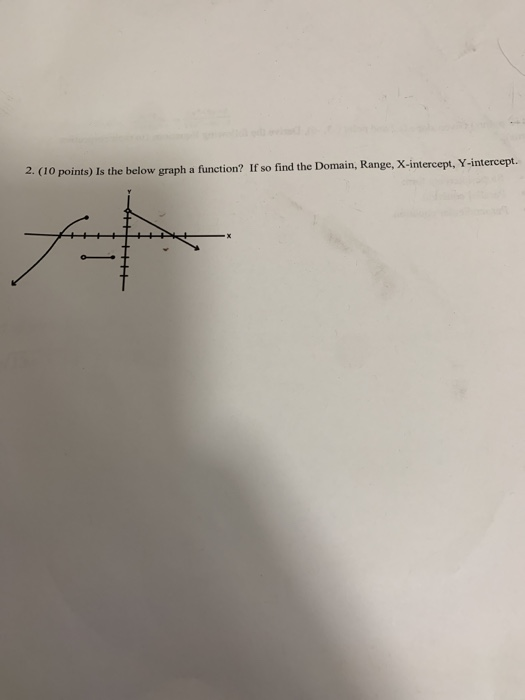2. (10 points) is the below graph a function? If so find the Domain, Range, X-intercept, Y-intercept. A

• ### 7. For each function below, find the intercept(s) (if any) and asymptote(s) (both horizontal and vertical,...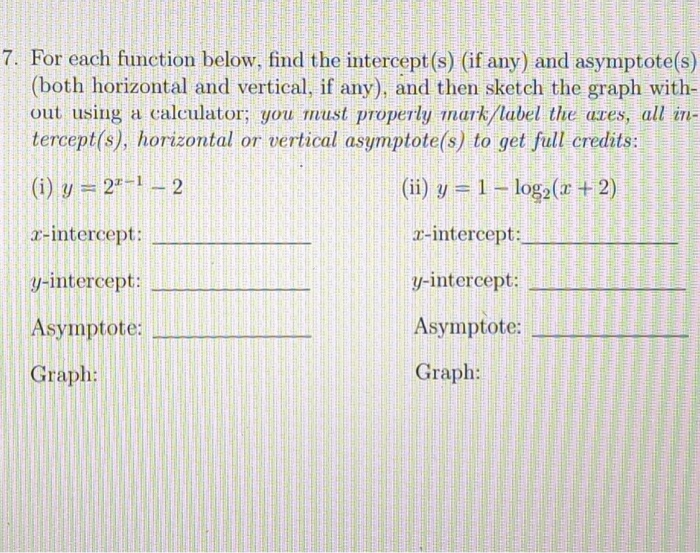7. For each function below, find the intercept(s) (if any) and asymptote(s) (both horizontal and vertical, if any), and then sketch the graph with- out using a calculator; you must properly mark/label the ares, all in- tercept(s), horizontal or vertical asymptote(s) to get full credits: (i) y = 22-1 – 2 (ii) y=1- log2 (x + 2) x-intercept: 2-intercept: y-intercept: y-intercept: Asymptote: Asymptote: Graph: Graph:

• ### holes, asymptopes, y intercept, zeros, domain, range

y= (x+1)/ x^2 + 3x + 2need to figure out what a graph looks likeis there holeswhat are the asymptotes if anywhat the zeros aredomain and rangewhere increasing decreasingy interceptnegative/positiveodd/ even or neitherI am so new to this and totally lost

• ### How do i solve the functions domain, range, x intercept and y intercept for this function

How do i solve the functions domain, range, x intercept and y intercept for this function..there is a a shaded dot on (-4,-4) and then theres a curved line going up to an unshaded dot on (0,0)

• ### I need to write 2 equations that represent the same exponential function with a y-intercept of 5 and an asymptote at y=3

I need to write 2 equations that represent the same exponential function with a y-intercept of 5 and an asymptote at y=3. I got y=2^(x+1) + 3 but I don't know how to find the second equation. Can someone please explain this to me. Thanks.

Free Homework App